# RS Aggarwal Solutions for Class 9 Chapter 3: Factorisation of Polynomials Exercise 3B

## RS Aggarwal Solutions for Class 9 Maths Exercise 3B PDF

This exercise has problems which are solved on Factorising the difference of two squares by the subject experts at BYJU’S. Our team of faculties mainly work with the aim of making Mathematics easier for students based on their grasping power.The students can gain a grip on the concepts covered in Maths Class 9 only by regular practise and while solving can make use of the PDF as reference. Solving the main problems will become easier if the students first solve the examples which are present before each exercise. RS Aggarwal Solutions for Class 9 Maths Chapter 3 Factorisation of Polynomials Exercise 3B are provided here.

## RS Aggarwal Solutions for Class 9 Chapter 3: Factorisation of Polynomials Exercise 3B Download PDF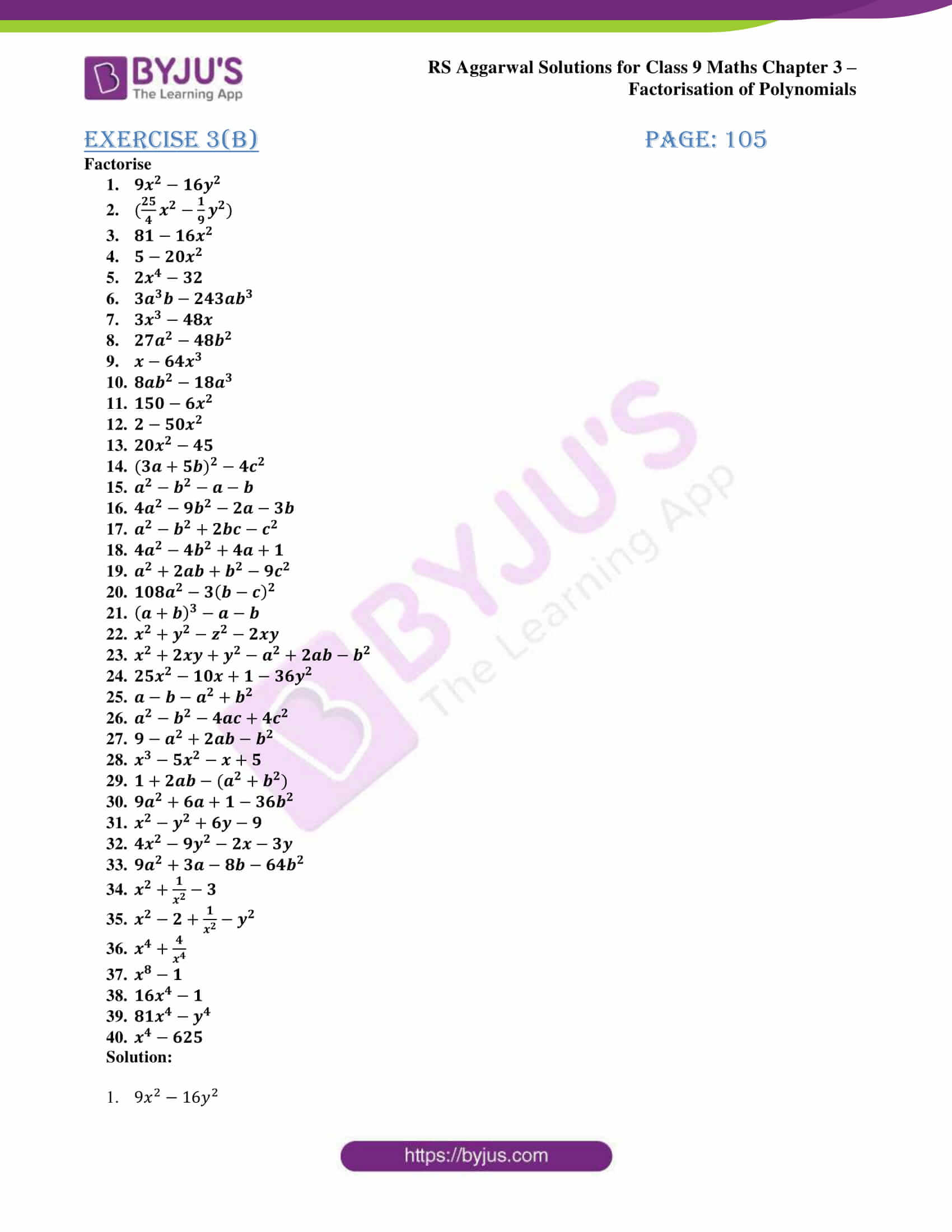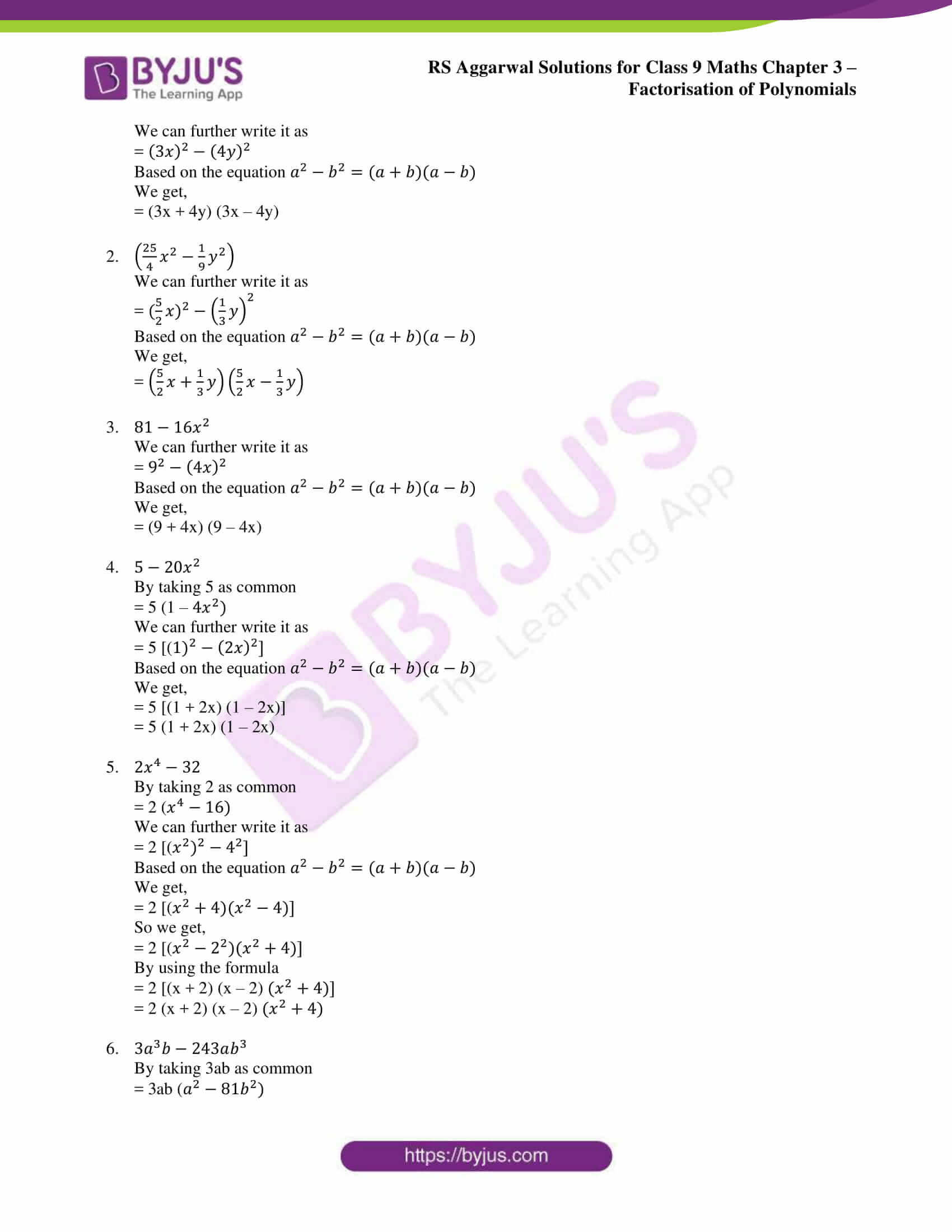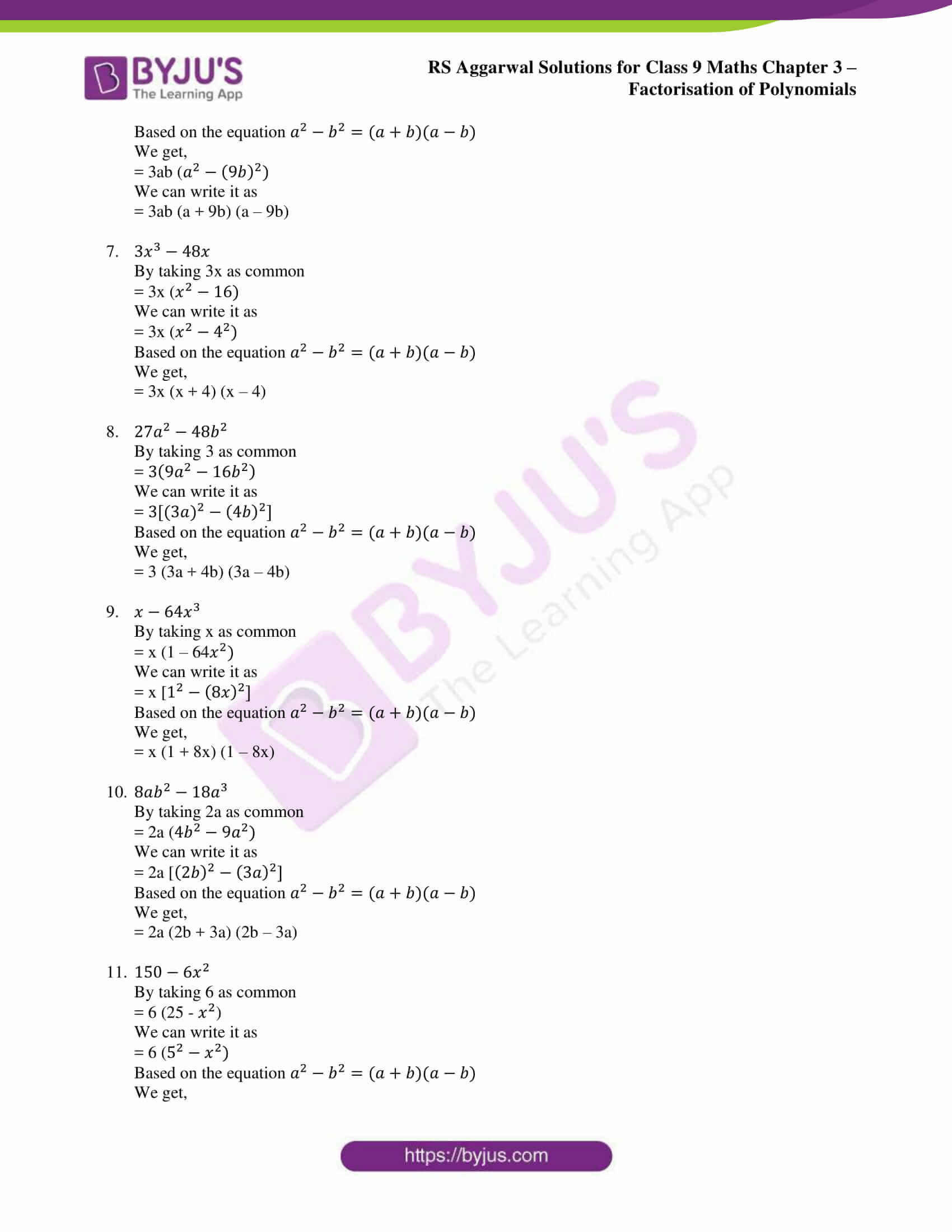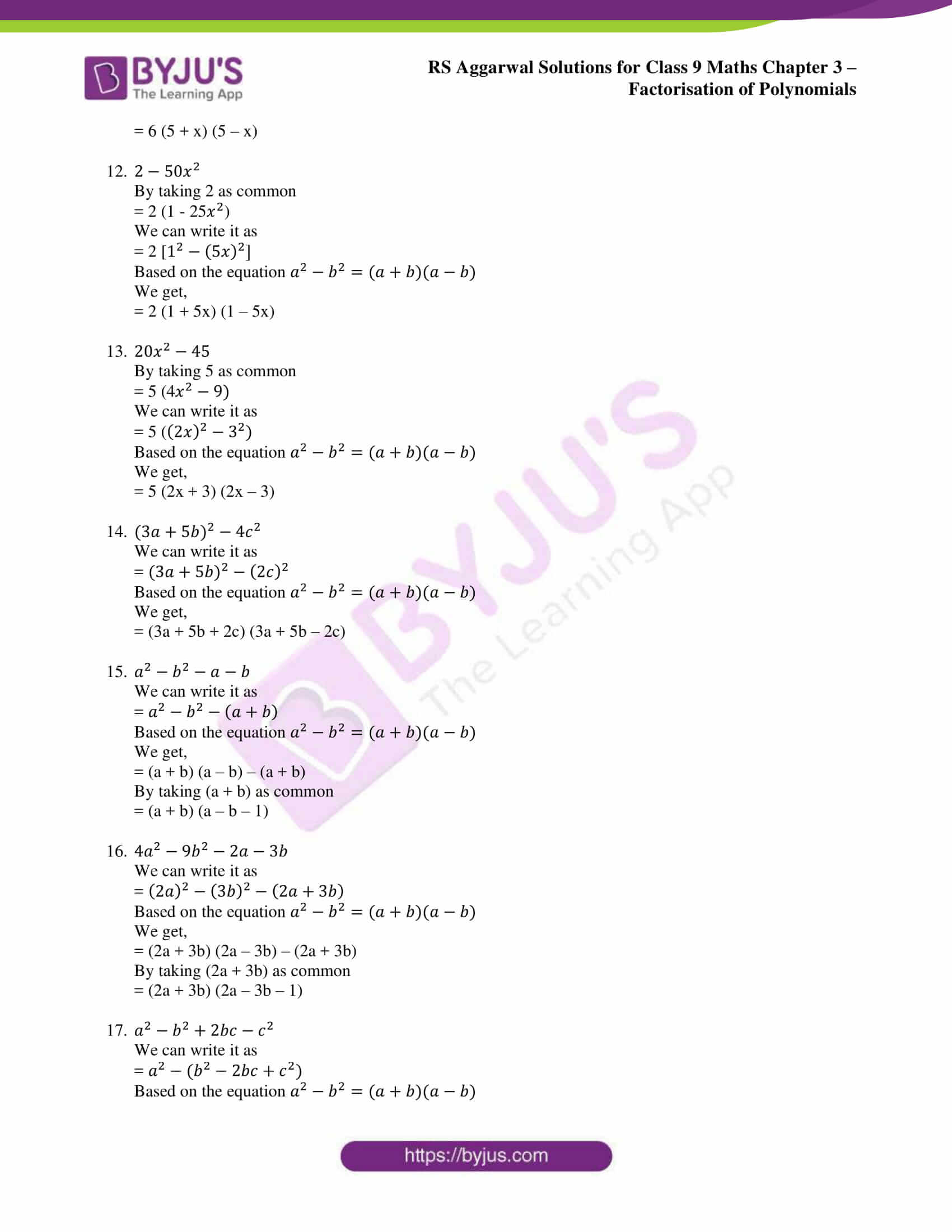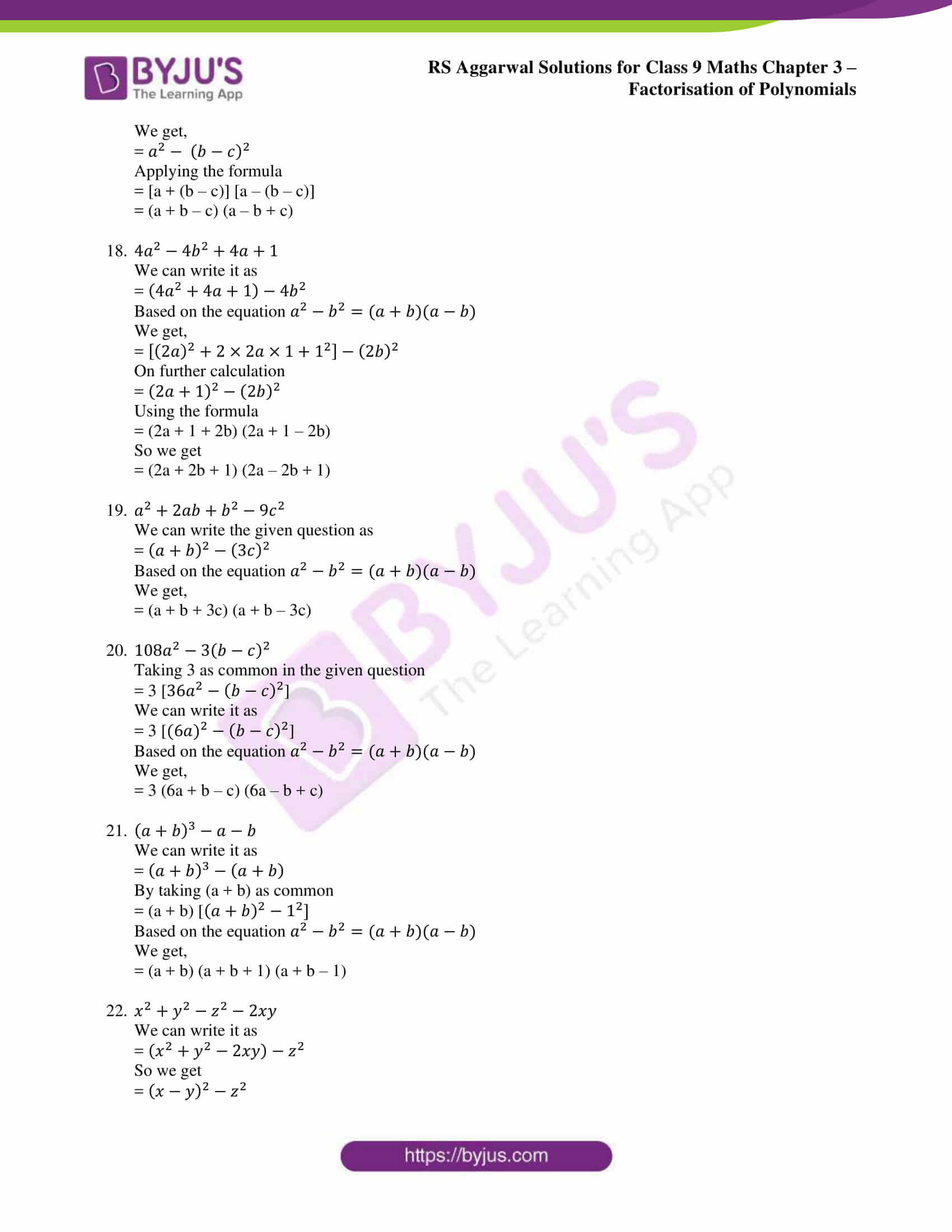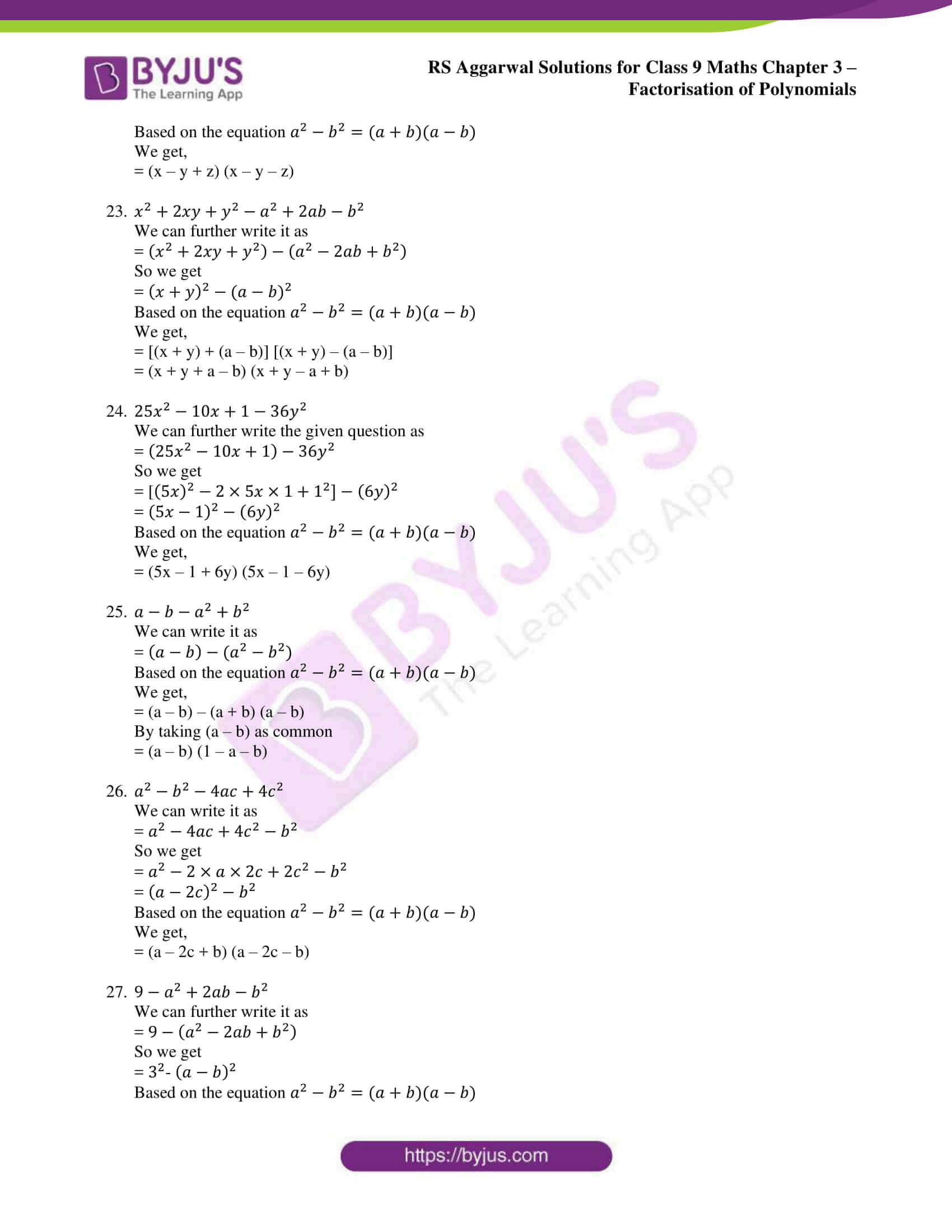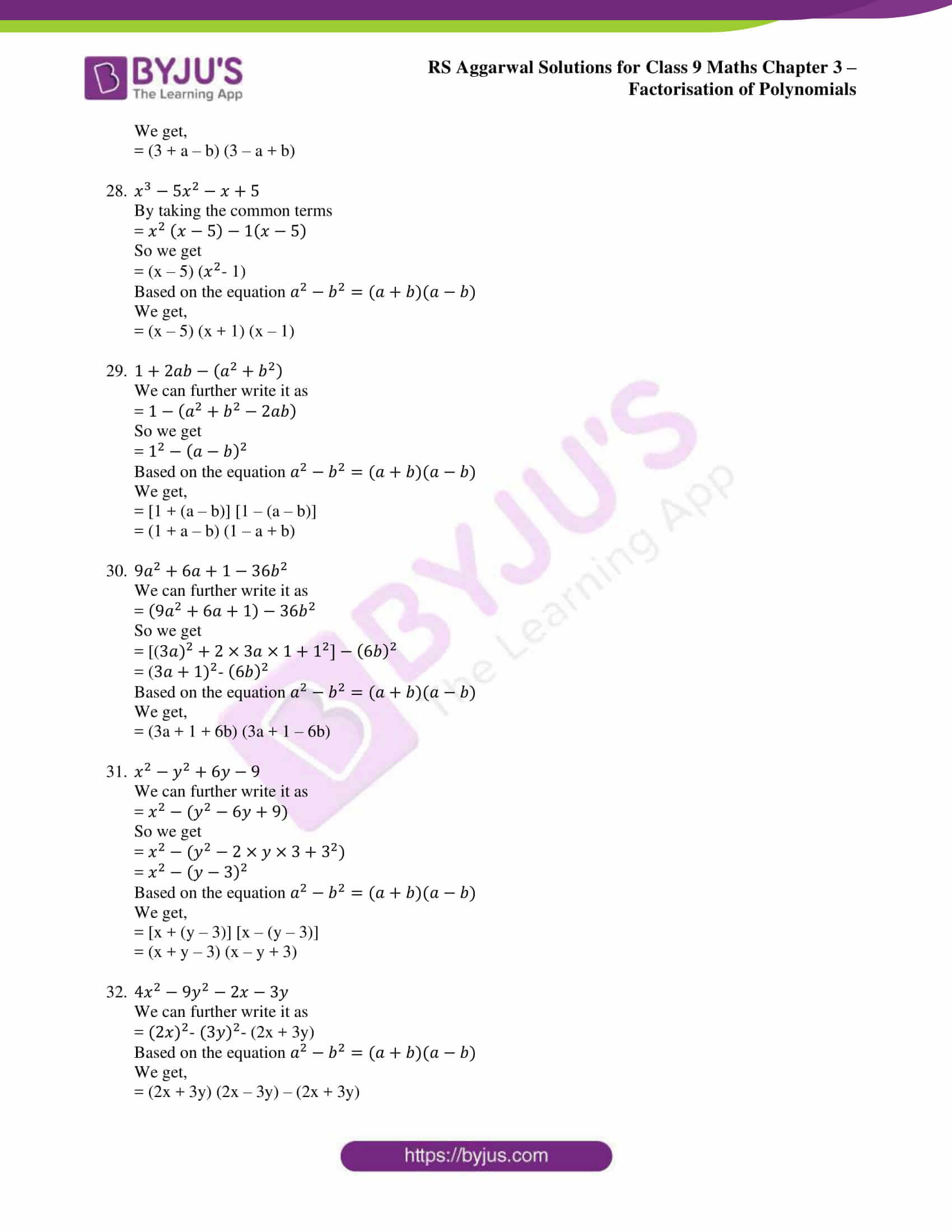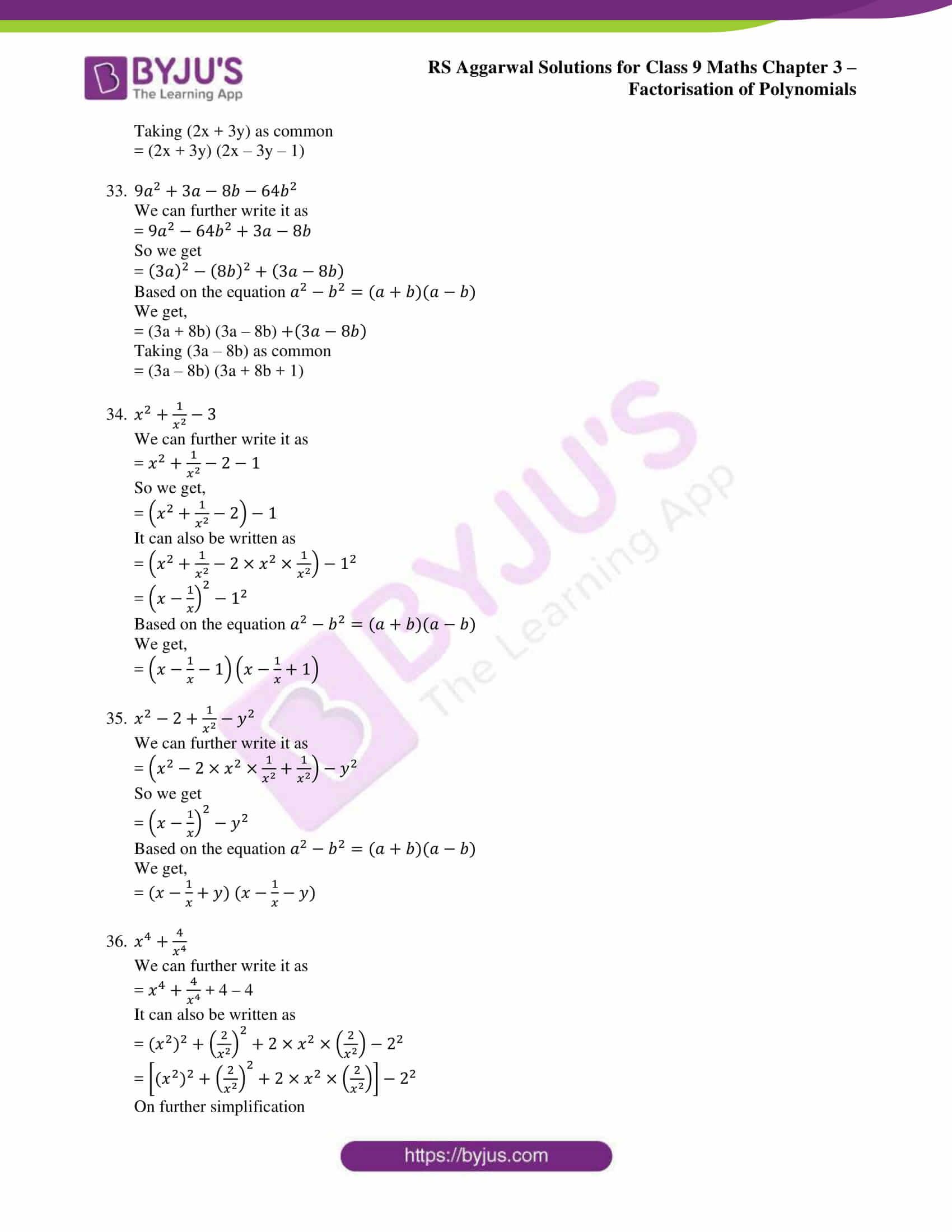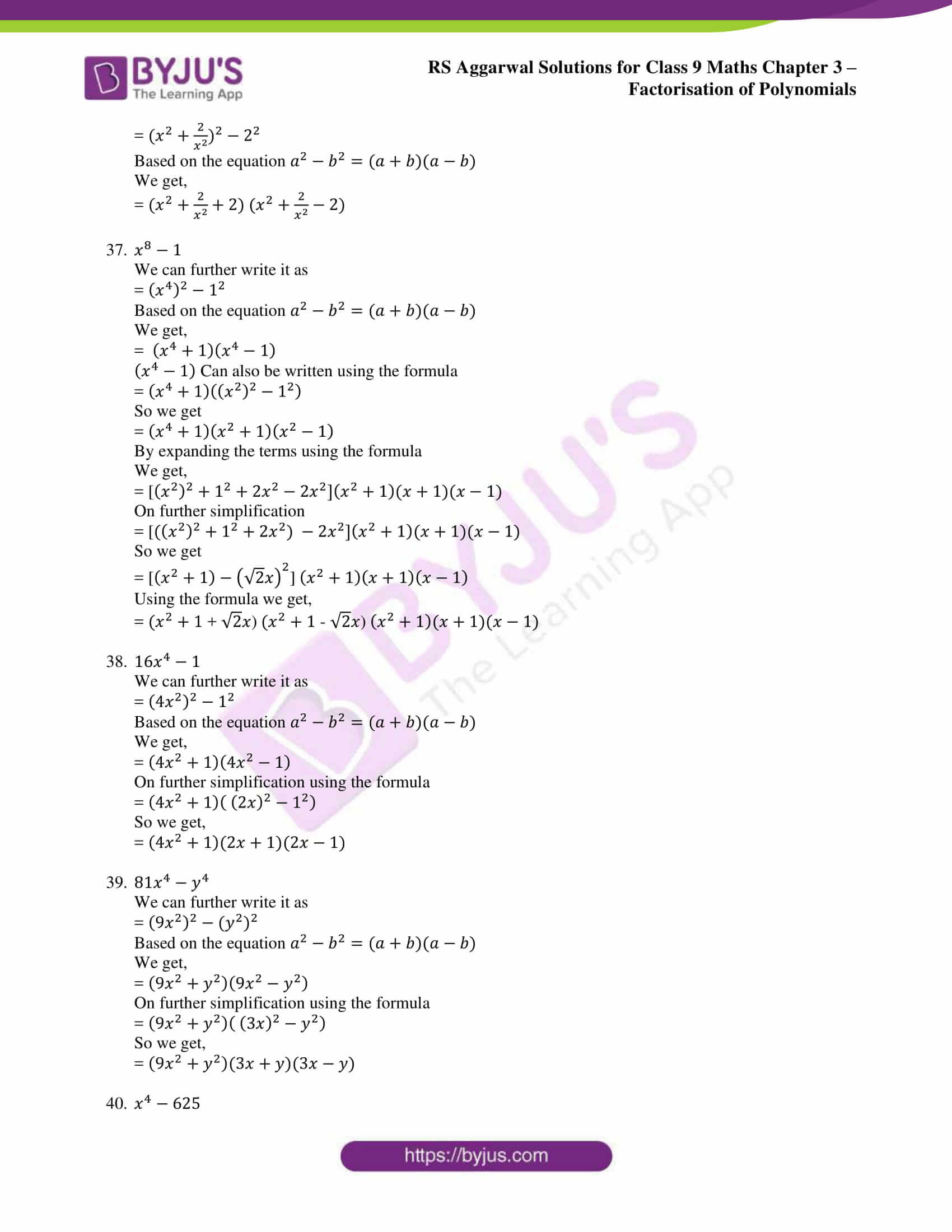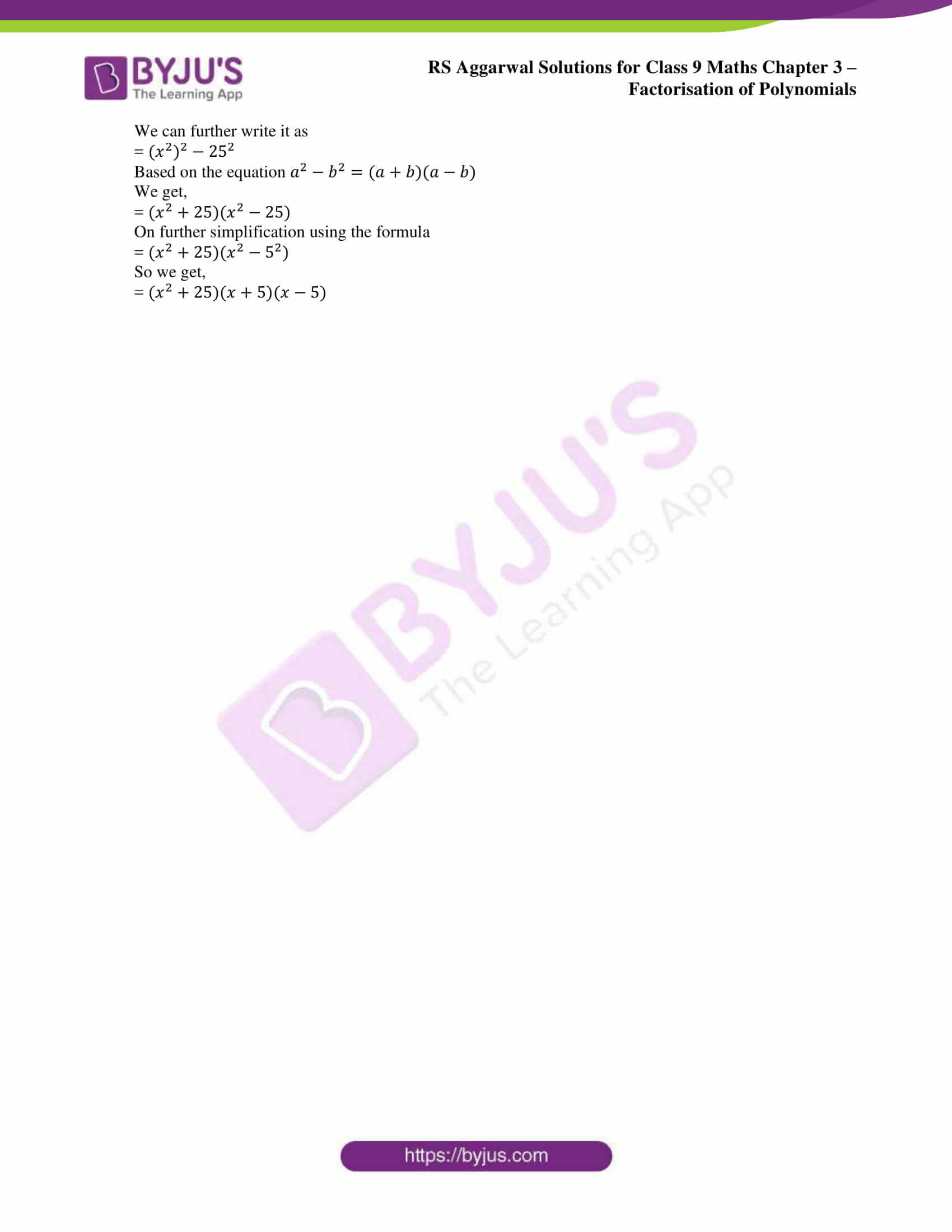### Access other exercise solutions of class 9 Maths Chapter 3: Factorisation of Polynomials

Exercise 3A Solutions 34 Questions

Exercise 3C Solutions 66 Questions

Exercise 3D Solutions 7 Questions

Exercise 3E Solutions 10 Questions

Exercise 3F Solutions 38 Questions

Exercise 3G Solutions 25 Questions

### RS Aggarwal Solutions Class 9 Maths Chapter 3 – Factorisation of Polynomials Exercise 3B

RS Aggarwal Solutions Class 9 Maths Chapter 3 Factorisation of Polynomials Exercise 3B has examples and problems which are solved based on the concept of Factorising the difference of two squares.

### Key features of RS Aggarwal Solutions for Class 9 Maths Chapter 3: Factorisation of Polynomials Exercise 3B

• Students gain a deep understanding of the concepts, which are based on the RS Aggarwal textbook of CBSE syllabus.
• RS Aggarwal solutions has problems solved in PDF format for all the exercises in each chapter.
• The students while solving the chapter for exam preparation can use this as a guide to identify the mistakes they do regularly and correct them.
• Difficult problems can be solved easily by the students by referring RS Aggarwal solutions.